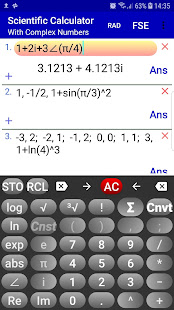#Complex Number Calculator | Polar Complex Calc v1.3.7 [Paid]

Complex Number Calculator | Polar Complex Calc v1.3.7 [Paid]
Requirements: 4.0.3 and up
Overview: Feature-Rich, Easy-to-Use Complex Number Calculator. Easy-to-use Scientific Calculator with a unique user interface and useful features.[break]

Use this complex number calculator as a scientific calculator. Any operation or function evaluation you can do with real numbers, you can also do the same with complex numbers by using this complex calculator. Namely, calculate expressions containing real, imaginary and, in general, any complex number in any form including, but not limited to, the standard (rectangular) and polar forms.

This complex calculator lets you enter complex numbers in the standard (rectangular) or in the polar form. The calculated values will be displayed in standard form; optionally, this complex calculator displays the results in the polar form and other modular forms.

With a unique intuitive user interface this scientific calculator, being a comprehensive complex number calculator, computes arbitrary complicated complex number expressions with exceptional ease and converts complex numbers to polar form.

 Supports all standard mathematical functions with real, imaginary and complex numbers as their arguments including trigonometric, exponential, logarithmic, hyperbolic and inverse trig/hyperbolic functions plus the Gamma function, Γ, Psi function, Ψ and zeta function, ζ.

Swipe the number pad to the right to see more functions (trig, hyperbolic, inverses, etc.).

 Calculate expressions containing complex numbers in standard form a+bi and polar (phasor) form r∠(θ).

 Convert complex numbers from rectangular to polar and other modular forms and vice versa (long press i or ∠ to see all forms of the calculated result).

In addition to evaluating trig and related functions, this scientific complex number calculator allows the angle of polar form of complex numbers to be entered in radians or degrees.

 Fixed, scientific and engineering notations.

This scientific calculator also let you do statistics with one or two dimensional real data:
 x1, x2 ,x3, ... .
 Press Σ to calculate sum, mean, max, min, variance, sample and standard deviation, median, upper and lower quartile, etc.

 x1, y1; x2, y2; x3, y3; ... .
 Press Σ to calculate the equation of the linear regression line and graph it.

 Long press ! to calculate combinations C(n, r) and permutations P(n, r).

 Enter functions f(x) or parametric equations p(t) and generate table of values.

 Physical/Chemical/Atomic and other scientific constants.

 Easy to use unit converter (Time, Mass, Length, Velocity, and many more).

All of the above with more details are included in the built-in Instruction menu accompanying this scientific complex calculator.

[Login or [Only Registered and Activated users can see Content.]

What's New:
Performance improvement and some tweaks.
Now, the keypad buttons have a new shape. You can switch to the previous look: go to "Look & Feel" and check "Use round corners" for keypad buttons ("Color setting" is changed to "Look & Feel").

If crashed on update uninstall and install again.

``https://play.google.com/store/apps/details?id=com.gcalcd.calculator.scientific&hl=en``